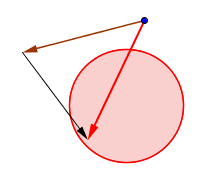# Complex NumbersHenri Picciotto

## Introduction

During my many years teaching high school math, I developed a graphical approach to the teaching of complex numbers, geared to Algebra 2 students, and inspired by the now out-of-print Algebra II / Trigonometry: A Guided Inquiry, by Chakerian, Stein, and Crabill. See a fun kinesthetic introduction to this, by Michael Pershan and Max Ray, here.

The basic idea is to start by defining complex numbers as (corresponding to) points in the plane, which can be thought of in rectangular form (with normal Cartesian coordinates,) or in polar form. These numbers have their own arithmetic: addition works like vector addition, and multiplication is defined as multiplying the r's and adding the θ's. After verifying that arithmetic for numbers on the x-axis (the real numbers) still work as expected, we find that in this system, -1 has a square root. We call it i.

That the approach makes sense need not be proved formally at the Algebra 2 level, but the proof is accessible to students in a later elective course, such as Precalculus, or my Space course. In Algebra 2 it is sufficient to confirm in several cases that the distributive rule in a+bi form yields the same result as the geometric multiplication in polar form.

The advantages of this approach are many.
- It provides some review and practice of basic trig.
- It gives a visual, concrete interpretation to complex numbers.
- It lays a foundation for the formulas for the sine and cosine of a sum, and the double angle formulas.
- It prepares students for the calculation of images of points under geometric transformations, including the rotation matrix.

## Games

These online games help provide a solidly intuitive introduction to complex number arithmetic.
Subtraction (rectangular form)
Multiplication (polar form)
Division (polar form)
(It's easy to cheat to improve your score, but that wouldn't do much to help you understand complex number arithmetic, now, would it.)

## Worksheets

From most basic to most advanced:
Kinds of Numbers (a worthwhile conversation starter)
Complex Numbers in Algebra 2
Complex Numbers, Transformations, and Matrices
— (classroom-tested, includes the proof that this approach makes sense,
— and some activities involving the TI-89 calculator)
GeoGebra version (not tested, but possibly useful)Related pages on this site:
Signed Number Arithmetic Games
Seeking Depth in Algebra 2
Space
Transformational Geometry
Applets Directory